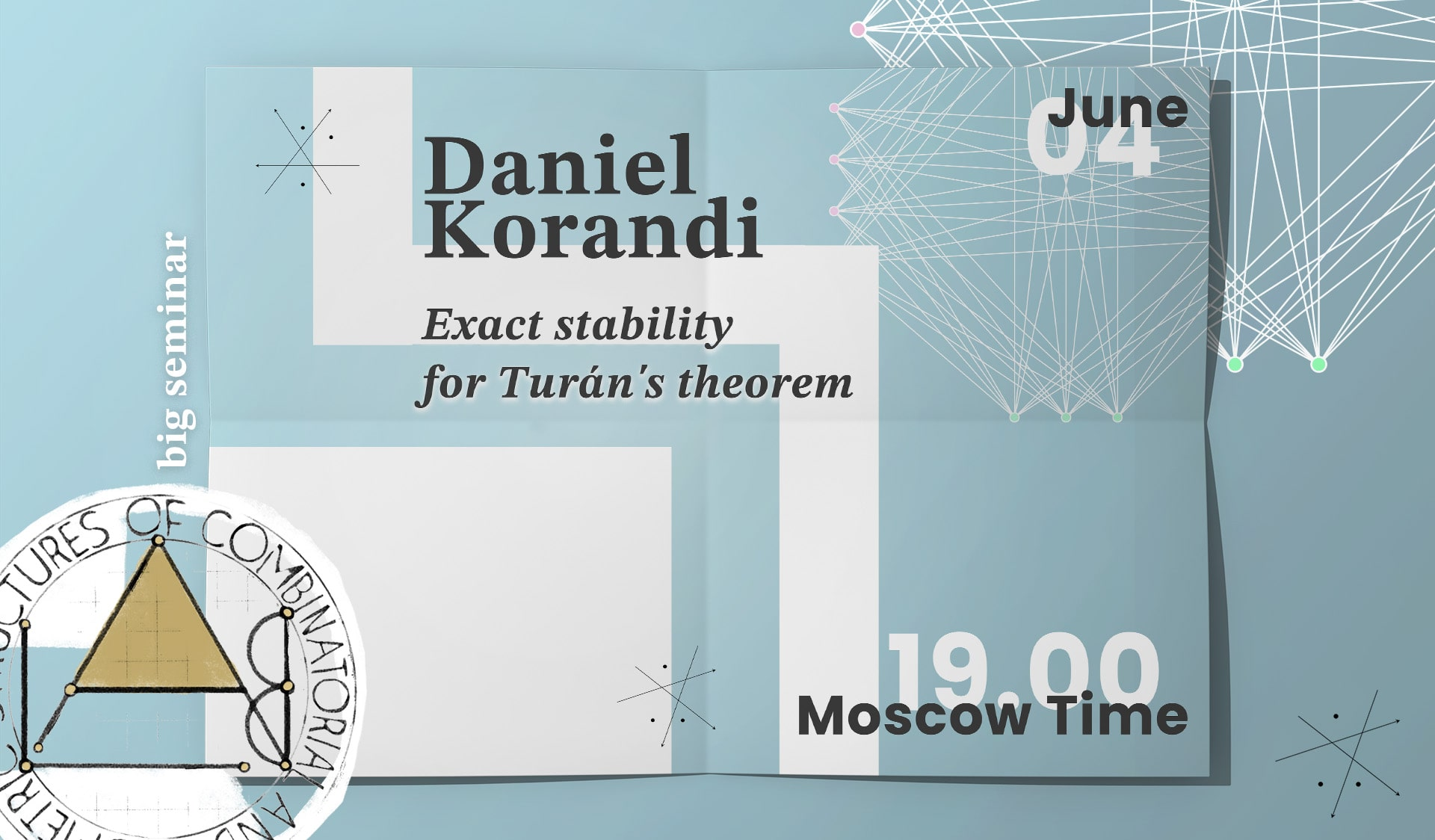# Daniel Korandi “Exact stability for Turán’s theorem”June 4, 2020
19.00 MSK (UTC +3)

# Daniel Korandi "Exact stability for Turán's theorem"

Daniel Korandi from Oxford University will give the talk "Exact stability for Turán's theorem" on the labs' Big Seminar.

The talk will be held in zoom
Meeting ID: 279-059-822
Password: first 6 decimal places of $\pi$

You can also write to Alexander Polyanskii (alexander.polyanskii@yandex.ru) or to Maksim Zhukovskii (zhukmax@gmail.com) if you want to be added to mailing list.

Abstract:

Turán's theorem says that an extremal $K_{r+1}$-free graph is $r$-partite. The Stability Theorem of Erdös and Simonovits shows that if a $K_{r+1}$-free graph with $n$ vertices has close to the maximal $t_r(n)$ edges, then it is close to being r-partite. In this talk we determine exactly the $K_{r+1}$-free graphs with at least m edges that are farthest from being r-partite, for any $m > t_r(n) - δn^2$. This extends work by Erdös, Györi and Simonovits, and proves a conjecture of Balogh, Clemen, Lavrov, Lidický and Pfender. Joint work with Alexander Roberts and Alex Scott.

## Watch the video:

Everyone is invited to attend. The language of the lecture is English. The event is aimed at master and graduate students, as well as researchers in the field of combinatorics.